• 两点之间最短距离 这是我的一个测试，也是我学习html的起点，他们说一个合格的程序员必须学会html，我比他们起步晚了一些，可是我认为还来的及，以后我就用html来记录我的学习记录了。 问题的提出...
    两点之间最短距离

这是我的一个测试，也是我学习html的/起点，他们说一个合格的程序员必须学会html，我比他们起步晚了一些，可是我认为还来的及，以后我就用html来记录我的学习记录了。

问题的提出：
在二维平面的n个点上，找出其中的一对点，使得在n个点组成的所有的点中，该点对的距离最小。

一维：最接近点对问题
方法一：（暴力法）
直接使用双层遍历来计算每一对点的距离，另外新建一个变量记录两点之间距离最小的值，但是如此一来时间复杂度就变为O(n2),

方法二：(分治法）
分治法的思路:

划分成子规模点集S1和S2；
找到S1和S2的最小距离d；
合并S1和S2：利用d在S1的(m-d,m]和S2(m,m+d]中找到最大点和最小点，即p3和q3.选出最小距离，完成合并。

m如何选择
m=(max(s)+min(s))/2;

代码：talking is cheap,show me the code;
/*根据算法随便写的，返回的是两个点之间最小的距离，不是两个点的坐标，如果有问题，欢迎指正*/
/*这里的坐标默认为正序的*/
#include<iostream>
#include<vector>
using namespace std;
int search(vector<int> target)
{
int n=target.size();
if(n<2)   //如果分到一个元素就返回最大数值
return INT_MAX;
int mid=n/2;   //中线
vector<int> temp_left;
vector<int> temp_right;   temp_left.insert(temp_left.begin(),target.begin(),target.begin()+mid); //中线分割  temp_right.insert(temp_right.begin(),target.begin()+mid,target.end());
int p=search(temp_left);
int q=search(temp_right);
int d=temp_right.front()-temp_left.back();
cout<<temp_right.front()<<" "<<temp_left.back()<<endl;
return d>min(p,q)?min(p,q):d;}//返回两边和贯穿中间的最小的两点距离
int main(){
int a={-9,-6,0,4,7,9};
vector<int> target(a,a+6);
cout<<search(target);
return 0;
}

二维最接近点的问题：
思路与一维的最接近点对相似。

选取中垂线l：x=m来作为分割直线。
递归的在S1和S2找出其最小距离d1和d2，并设d=min{d1,d2}，S中最接近点对或是d，或者是某个{p，q}
合并起来

// 随便写思路的如果有误，请指正（注意返回的是两点之间的距离，不是坐标）
#include <iostream>
#include <math.h>
#include<algorithm>
using namespace std;
struct point
{
int x, y;
};
double distance(struct point p1, struct point p2)
{
return sqrt(pow(p1.x - p2.x, 2) + pow(p1.y - p2.y,2));
}
double search(struct point p[],int pl,int pr)
{
if (pl == pr)
return INT_MAX;
int mid = (pl + pr) / 2;
double d_left = search(p, pl, mid);
double d_right = search(p, mid + 1, pr);
double temp_d = min(d_left, d_right);
while (abs(p[pl].y - p[mid].y) > temp_d&&pl < pr)//如果仅仅是单边距离就已经大于上一次比较返回的d就没必要在接下来的敌方继续比较了。
pl++;
while (abs(p[pr].y - p[mid].y) > temp_d&&pl < pr)
pr--;
for (int i = pl; i < pr; i++)
{
for (int j = i + 1; j < pr; j++)
{
if (temp_d < abs(p[i].x - p[j].x))//同理与上面的注释
break;
else
temp_d = min(temp_d, distance(p[i], p[j]));//找到最小的距离
}
}
return temp_d;
}
int main()
{
struct point p;
int n;
cin >> n;
for (int i = 0; i < n; i++)
{
cin >> p[i].x;
cin >> p[i].y;
}
cout<<search(p, 0, n - 1);
}




展开全文• 无向图两点之间最短距离怎么求？有什么算法可以实现啊。头疼死了，好几天了，求指点，给个具体点的代码。c语言
• 这一类 找两点间最短距离的问题一般是使用BFS来解决。 题目描述 给定一个矩阵，x 为坐标起点，即可以用作出发的地方，T 为终点， 即要到达的地方，那么请给出 从 X 出发能到达 T 的最短的路径长度， 以及起点 X 的...
找指定两坐标间的最短距离
这一类 找两点间最短距离的问题一般是使用BFS来解决。
题目描述
给定一个矩阵，x 为坐标起点，即可以用作出发的地方，T 为终点， 即要到达的地方，那么请给出 从 X 出发能到达 T  的最短的路径长度， 以及起点 X 的坐标。
输入：矩阵地图
5 6
X00100
00000X
01T000
0X1010
00000X

输出：一行是最短的路径距离，接着一行是起始点 X 的坐标。
4
0 0 1 5

思路
考虑 BFS
再说一次，广度优先搜索可回答两类问题。
 第一类问题：从节点A出发，有前往节点B的路径吗？（在你的人际关系网中，有芒果销
售商吗？）
 第二类问题：从节点A出发，前往节点B的哪条路径最短？（哪个芒果销售商与你的关系
最近？）
【算法图解】
代码
import java.util.*;

// 第二题
/*
5 6
X00100
00000X
01T000
0X1010
00000X

4
0 0 1 5
*/
public class FindShortestPath {
public static void main(String[] args) {
Scanner sc = new Scanner(System.in);
char[][] board;
PddNo2 pdd = new PddNo2();
while (sc.hasNext()){
String[] nm = sc.nextLine().split(" ");
int N = Integer.valueOf(nm);
int M = Integer.valueOf(nm);
board = new char[N][M];
for (int i = 0; i < N; i++) {
board[i] = sc.nextLine().toCharArray();
}

Map<Integer, List<Integer>> res = pdd.getRes(board);
Set<Map.Entry<Integer, List<Integer>>> entries = res.entrySet();

Iterator it = entries.iterator();
//while (it.hasNext()){
Map.Entry<Integer, List<Integer>> myres = (Map.Entry<Integer, List<Integer>>) it.next();
System.out.println(myres.getKey());
List<Integer> list = myres.getValue();
for (int i = 0; i < list.size(); i++) {
System.out.print(list.get(i));
if(i!=list.size())
System.out.print(" ");
}
System.out.println();
//            }
}
}
}

class PddNo2 {
public char[][] board;
public int N, M;
//    public boolean[][] marked;
public Map<Integer, List<Integer>> res;
public int[][] directions = {{0, 1}, {0, -1}, {1, 0}, {-1, 0}};
public int count = 0;

public Map<Integer, List<Integer>> getRes(char[][] board) {
if (board == null || board.length == 0 || board.length == 0)
return res;
// 默认升序排序
this.res = new TreeMap<>();
this.board = board;
this.N = board.length;
this.M = board.length;

List<Integer> list;
for (int i = 0; i < N; i++) {
for (int j = 0; j < M; j++) {
if (board[i][j] == 'X') {
//                    System.out.println("起点 "+" x -> "+i+" y -> "+j);
queue.clear();
list = BFS(queue, i, j);
if (list != null && list.size() != 0) {
int key = list.get(0).intValue();

if (res.containsKey(key)) {
List temp = res.get(key);
} else {
List<Integer> temp = new ArrayList<>();
res.put(key, temp);
}
}
}

}
}
return res;
}

// list 存储 [length, newX, newY] 表示 (i,j) 到 （newX, newY）的路径长度 length
public List<Integer> BFS(Queue<int[]> queue, int i, int j) {
// 这里必须要 创建新的 visited 访问表 因为 每一个起始点开始， 都是全新的路程 这里不会和之前的结果产生联系
boolean[][] visited = new boolean[N][M];
// 这个路径表 是用来记录每一 从 (i,j) 为起点的到自己这里的路径长度
// 使用一个新的路径长度表记录
int[][] gridLen = new int[N][M];
// 起始点到自己的 路径长度为 0
gridLen[i][j] = 0; // 起点

List<Integer> res = new ArrayList<>();
while (!queue.isEmpty()) {
// 移除队列头部的坐标
int[] xy = queue.remove();
// 作为起点
int length = gridLen[xy][xy];
visited[xy][xy] = true;
for (int k = 0; k < 4; k++) {
int newX = xy + directions[k];
int newY = xy + directions[k];

if (!inArea(newX, newY) || board[newX][newY] == '1' || visited[newX][newY])
continue;

gridLen[newX][newY] = length + 1;

if (board[newX][newY] == 'T') {
return res;
}
}
}
return null;
}

public boolean inArea(int x, int y) {
return x >= 0 && x < N && y >= 0 && y < M;
}
}



展开全文算法 java 数据结构 BFS
• // first是最短距离，second是顶点编号 bool vis[N]; vector G4[N]; void build(); // 建图 void dijkstra( int s, vector G[N], int *preV); // 求最短路径 void solve(); // 主体 ...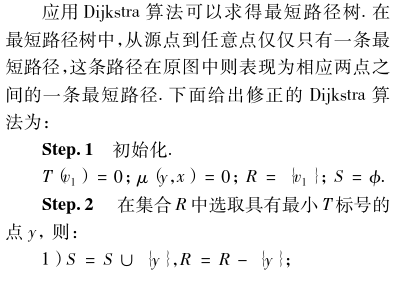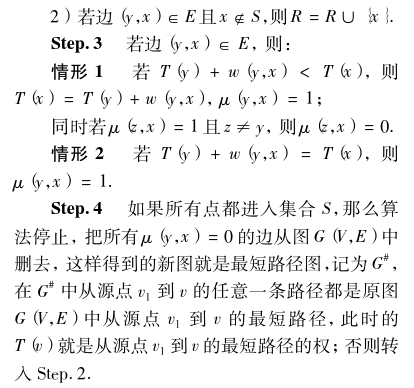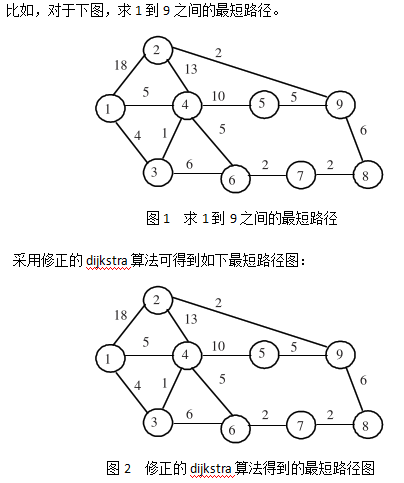#include "iostream"
#include "string"
#include "fstream"
#include "vector"
#include "queue"
#include "sstream"
#include "set"
#include "string.h"
#include "math.h"
#include <functional>

using namespace std;

#define INF 200000000
#define N 1000

struct Edge
{
int to;          // 边终止节点
int cost;        // 花费

Edge(int to1, int cost1)
{
to = to1;
cost = cost1;
}
};

int nV;                      // 顶点数
int nE;                      // 边数
vector<Edge> G[N];           // 图的邻接表形式
int G1[N][N];                // 图的邻接矩阵形式
int dist[N];                 // 从源点出发的最短距离
typedef pair<int, int> P;    // first是最短距离，second是顶点编号
bool vis[N];
vector<Edge> G4[N];

void build();                                                  // 建图
void dijkstra(int s, vector<Edge> G[N], int *preV);            // 求最短路径
void solve();                                                  // 主体

void addEdge(int from, int to, int cost, vector<Edge> G[N])
{
Edge e(to, cost);
G[from].push_back(e);

Edge e1(from, cost);
G[to].push_back(e1);
}

void build()
{
int i;
ifstream fin;
fin.open("data.txt");
cout << "顶点数：";
fin >> nV;
cout << nV << endl;
cout << "边数：";
fin >> nE;
cout << nE << endl;

// 输入图
for(i=0; i<nV; i++)
{
for(int j=i; j<nV; j++)
{
G1[i][j] = G1[j][i] = INF;
}
}
cout << endl << "原图{边的起点，终点，花费}："  << endl;
int from, to, cost;
for(i=0; i<nE; i++)
{
fin >> from >> to >> cost;
cout << from << " " << to << " " << cost << endl;
G1[from][to] = G1[to][from] = cost;
}
fin.close();
}

void dijkstra(int s, vector<Edge> G[N])
{
fill(dist, dist + nV+1, INF);
priority_queue<P, vector<P>, greater<P> > q;
dist[s] = 0;
q.push(P(0, s));
while(!q.empty())
{
P p = q.top();   //从尚未使用的顶点中找到一个距离最小的顶点
q.pop();
int v = p.second;
if(dist[v] < p.first)
continue;
for(int i=0; i<G[v].size(); i++)
{
Edge &e = G[v][i];
int dis = dist[v] + e.cost;
if(dist[e.to] > dis)
{
dist[e.to] = dist[v] + e.cost;
q.push(P(dist[e.to], e.to));
G4[v].push_back(e);
}
else if(dist[e.to] == dis)
{
G4[v].push_back(e);
}
}
}
}

struct Ans
{
vector<int> path;
int cost;
int start;

void getCost()
{
cost = G1[start][path];
for(int i=0; i<path.size()-1; i++)
{
cost += G1[path[i]][path[i+1]];
}
}
};

void dfs(int s, int t, Ans &A, vector< Ans > &paths, int start)
{
if (s == t)
{
A.start = start;
A.getCost();
paths.push_back(A);
}

for (int i = 0; i < G4[s].size(); i++)
{
int u = G4[s][i].to;
if (!vis[u])
{
vis[u] = true;
A.path.push_back(u);
dfs(u, t, A, paths, start);
A.path.pop_back();
vis[u] = false;
}
}
}

void solve()
{
build();
dijkstra(1, G);

int i, j;
cout << endl << "最短路径图{边的起点，终点，话费}："  << endl;
for(i=0; i<nV; i++)
{
for(int j=0; j<G4[i].size(); j++)
{
cout << i << " " << G4[i][j].to << " " << G4[i][j].cost << endl;
}
}

vector<Ans> paths;
Ans ans;
memset(vis, false, sizeof(vis));
dfs(1, 9, ans, paths, 1);

cout << endl << "1到9的所有最短路径：" << endl;
for(i=0; i<paths.size(); i++)
{
cout << "1 ";
for(j=0; j<paths[i].path.size(); j++)
{
cout << paths[i].path[j] << " ";
}
cout << "---cost：" << paths[i].cost << endl;
}
}

int main()
{
solve();
getchar();
return 0;
}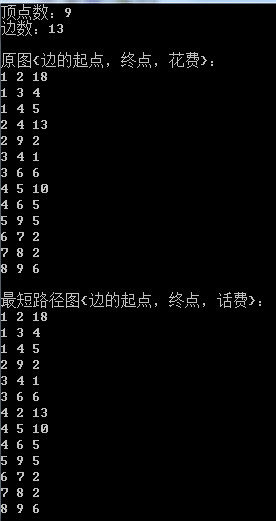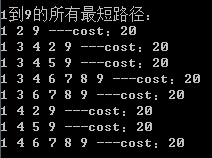参考文献 https://wenku.baidu.com/view/0254244b001ca300a6c30c22590102020740f2d8.html
王志坚，韩伟一，李一军. 具有多条最短路径的最短路问题[J]. 哈尔滨工业大学学报，2010，42（9）：1429-1431.

附
data.txt

9
13
1 2 18
1 3 4
1 4 5
2 4 13
2 9 2
3 4 1
3 6 6
4 5 10
4 6 5
5 9 5
6 7 2
7 8 2
8 9 6

展开全文• 经纬度一点与经纬度两点之间测最短距离思路代码 思路 如果有数学基础，看到这个图就应该懂了。 首先，拿到两个经纬度计算x,y轴各自的差值： 36.679430 - 36.633990 = xN 116.952210 - 117.054860 = yN 拿到x,y轴...
经纬度一点与经纬度两点之间测最短距离思路代码
思路如果有数学基础，看到这个图就应该懂了。
首先，拿到两个经纬度计算x,y轴各自的差值：
36.679430 - 36.633990 = xN
116.952210 - 117.054860 = yN
拿到x,y轴各自的差值之后，计算向下移动几个点位。平均分就可以，xN/4,yN/4(我只取3个中间点，所除以4，如果想要精细，这个除数越大越好)
那么，116.952210-yN1，36.679430-xN1就是第一个中间点坐标的经纬度。
116.952210-yN2，36.679430-xN2就是第二个中间点坐标的经纬度。
…
以此类推
每次拿到一个中间点，就可以计算他与线外点的距离。
拿到所有的中间点，放入list中。就可以用stream().min()方法获取距离的最小值。
好了，直接上代码
代码
/**
* @描述 反余弦进行计算，获取长度
* @参数  [lat1, lng1, lat2, lng2]
* @返回值  double
* @创建人  JiangWei
* @创建时间  2020/10/22 20:31
**/
public static double getDistance(Double lat1,Double lng1,Double lat2,Double lng2) {
// 经纬度（角度）转弧度。弧度用作参数，以调用Math.cos和Math.sin

// 公式中“cosβ1cosβ2cos（α1-α2）+sinβ1sinβ2”的部分，得到∠AOB的cos值
//        System.out.println("cos = " + cos); // 值域[-1,1]
double acos = Math.acos(cos); // 反余弦值
//        System.out.println("acos = " + acos); // 值域[0,π]
//        System.out.println("∠AOB = " + Math.toDegrees(acos)); // 球心角 值域[0,180]
return EARTH_RADIUS * acos; // 最终结果
}

/**
* @描述 将两个点之间练成的拆分成N个经纬度，并计算每个拆分点到查询点的距离，返回拆分点的经纬度以及距离。
* @参数  Map[lat, lng, lat1, lng1, lat2, lng2]
* @返回值  List
* @创建人  JiangWei
* @创建时间  2020/10/22 20:31
*/
public static LatLngEntity getShortestDistance(Map<String,Double> map) {
double lat = map.get("lat");//选点坐标纬度
double lat1 = map.get("lat1");//A点左边纬度
double lat2 = map.get("lat2");//B点坐标纬度
double lng = map.get("lng");//选点坐标纬度
double lng1 = map.get("lng1");//A点左边纬度
double lng2 = map.get("lng2");//B点坐标纬度
//先A点纬度-B点纬度。拿到上下移动单位数
double mobileUnitLat = lat1-lat2;
//先A点纬度-B点纬度。拿到左右移动单位数
double mobileUnitLng = lng1-lng2;
//除以11，分成10份，也就是10个经纬度的点。
DecimalFormat dFormat = new DecimalFormat("0.00000000");
String latDistance = dFormat.format(mobileUnitLat/21);
String lngDistance = dFormat.format(mobileUnitLng/21);
List<LatLngEntity> latLonList = new ArrayList<>();
for (int i = 1;i<=4; i++){
LatLngEntity latLngEntity = new LatLngEntity();
double latDistanceLat = lat1-Double.valueOf(latDistance)*i;//拿到线变点的第i个经度
double lngDistanceLng = lng1-Double.valueOf(lngDistance)*i;//拿到线变点的第i个纬度
Double distance =  getDistance(latDistanceLat,lngDistanceLng,lat,lng);
latLngEntity.setLat(latDistanceLat);
latLngEntity.setLng(lngDistanceLng);
latLngEntity.setDistance(distance);
}
LatLngEntity maxAgeUser = latLonList.stream().min(Comparator.comparing(LatLngEntity::getDistance)).get();
return maxAgeUser;
}



展开全文java
• 给定地球上两点的经纬度，计算...现在想求出A和B两点之间沿着地球表面最短的弧线距离(图中红线标出部分)。 思考：假设地球是个标准的球体（实际上地球是一个两极稍扁，赤道略鼓的椭球），很显然，只要知道A、B两点与机器学习实战 KMeans
• 按照象棋中马走的方向,判断两点最短距离或不可到达; http://poj.org/problem?id=2243 #include&lt;iostream&gt; #include&lt;queue&gt; #include&lt;cstring&gt; using namespace std;...
• using System; using System.Collections.Generic; using System.Linq; using System.Text;...//迪杰斯特拉算法 计算两点最短距离 namespace Dijkstra { class Program { /// /// 邻接矩阵 ///
• 基本思想 通过Floyd计算图G=(V,E)中各个顶点的最短路径时，需要引入一个矩阵S，矩阵S中的元素a[i][j]表示顶点i(第i个顶点)到顶点j(第j个顶点)的距离。 假设图G中顶个数为N，则需要对矩阵S进行N次更新。初始时，...学习算法
• #include #include #include #include ...#include //Floyd算法，求图中点之间最短距离 #define inf 1000000 //hdu2544 using namespace std; int n, map; int main() { int i, t
• （1）与迪杰斯特拉（Dijkstra）算法一样，弗洛伊德(Floyd)算法也是一种用于寻找给定的加权图中顶点间最短路径的算法，该算法 名称以创始人之一、1978 年图灵奖获得者、斯坦福大学计算机科学系教授罗伯特·弗洛伊德...
• （1）与弗洛伊德(Floyd)算法一样，迪杰斯特拉（Dijkstra）算法也是一种用于寻找给定的加权图中顶点间最短路径的算法，主要 特点是以出发为中心向外层层扩展（广度优先搜索思想），直到扩展到终点为止 2. 迪杰...
• 两点之间最短路径（Floyd算法）源代码 调试通过的
• 如果中转为K，距离矩阵为D 当D(i,K)+D(K,j)(i,j) 则D(i,j)=D(i,K)+D(K,j) 否则不变 程序的参数说明 P为最短路，顶点以经过次序排序 u为最短距离 W为距离矩阵 k1为起始 k2为终止 MATLAB实现 ...
• 不过路多了也不好，每次要从一个城镇到另一个城镇时，都有许多种道路方案可以选择，而某些方案要比另一些方案行走的距离要短很多。这让行人很困扰。 #include&lt;cstdio&gt; #include&lt;cstring&...
• 求三个维度中个向量 O 和 V 之间最短（垂直）距离。 Q 是连接 O 和 V 的向量。还需要知道每个向量上的一个来计算 Q (Q=Point1-Point2) SD 是函数返回的最短距离。 角度是个向量之间的角度。 例子： O = ...matlab
• 我对弗洛伊德算法的理解(求图中任意两点之间最短距离) 基本过程： for k = 1:n for i = 1:n for j = 1:n if route(i,k)+route(k,j) (i,j) route(i,j) = route(i,k) + route(k,j);
• 求从P经过A、B、C、D、E、F、G、H、I后到达F，不必考虑这9个的相对顺序，求P到J的最短路径，
• 给定平面上的至少n个（n〉=20），找出其中的一对，使得在n个组成的所有对中，该对间的距离最小。
• 题目已经讲述查找输入点与点之间的最小距离，特别需要注意的是 可能存在多个点对都符合最短距离要求，因此需要全部输出； 比如(1,1) (2,2) (3,3) 这里就有对点都符合(1,1) (2,2) 和 (2,2) (3,3) 二、代码 注意： ...java 数据结构
• 两点顶点之间最短路径问题 基于matlab的dijkstra算法 前言 看了很多篇介绍dijkstra算法的博客，没发现对于程序来说很多的地方都有问题。 一、函数代码 代码如下（示例）： function [ mydistance,mypath] = ...dijkstra 算法
• Dijkstra算法是求源点到其它顶点的最短路径。怎样求任意个顶点之间最短路径？
• 在计算两点之间最短路径时，如果两点之间存在其他的点，那么可以将最短路径的情况分为两类，经过某个点和不经过这个点。那么在求有向图中ab两点的最短路径时，遍历剩下的点，比较在a到b的路径中是经过Vi距离短...算法
• //邻接矩阵记录两点之间距离 int used; //标记经过的点 int sum; //记录从起点到所有终点的最短距离。 int len; //累次叠加的长度 int main() { int n,m,i,j,x,y,time,temp,min,record; while( ...output input 算法 c...